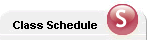Carleton.ca People

##Courses# Mathematics (MATH)

## School of Mathematics and StatisticsFaculty of Science

Note:

Consult the regulations concerning Deferred Examinations in the Academic Regulations section of this Calendar.

Prerequisites for First-year Mathematics Courses in B.Math. Programs

Students who do not have the required Ontario Grade 12 Mathematics courses or equivalents may take MATH 0005 in lieu of Advanced Functions, MATH 0007 and MATH 0107 in lieu of Calculus and Vectors. These 0000-level mathematics courses serve as alternate prerequisites for MATH 1002 and MATH 1102. These courses would be in addition to the minimum 15.0 credits required in General programs, or 20.0 credits required in Honours programs.

MATH 0005 - Precalculus: Functions and Graphs
MATH 0007 - Introductory Calculus
MATH 0107 - Algebra and Geometry
MATH 1002 - Calculus and Introductory Analysis I
MATH 1004 - Calculus for Engineering or Physics
MATH 1005 - Differential Equations and Infinite Series for Engineering or Physics
MATH 1007 - Elementary Calculus I
MATH 1009 - Calculus: with Applications to Business and Economics
MATH 1102 - Algebra I
MATH 1104 - Linear Algebra for Engineering or Science
MATH 1107 - Linear Algebra I
MATH 1119 - Linear Algebra: with Applications to Business and Economics
MATH 1401 - Elementary Mathematics for Economics I
MATH 1402 - Elementary Mathematics for Economics II
MATH 1805 - Discrete Structures I
MATH 2000 - Calculus and Introductory Analysis II (Honours)
MATH 2004 - Multivariable Calculus for Engineering or Physics
MATH 2007 - Elementary Calculus II
MATH 2008 - Intermediate Calculus
MATH 2009 - Intermediate Calculus for Science Students
MATH 2100 - Algebra II (Honours)
MATH 2107 - Linear Algebra II
MATH 2108 - Abstract Algebra I
MATH 2200 - Co-operative Work Term Report 1 (Honours)
MATH 2210 - Introduction to Geometry
MATH 2404 - Ordinary Differential Equations I
MATH 2454 - Ordinary Differential Equations (Honours)
MATH 2800 - Discrete Mathematics and Algorithms
MATH 2907 - Directed Studies (Honours)
MATH 3001 - Real Analysis (Honours)
MATH 3002 - Calculus of Differential Forms and Geometry (Honours)
MATH 3007 - Functions of a Complex Variable
MATH 3008 - Ordinary Differential Equations (Honours)
MATH 3009 - Introductory Analysis
MATH 3057 - Functions of a Complex Variable (Honours)
MATH 3101 - Algebraic Structures with Computer Applications
MATH 3106 - Introduction to Group Theory (Honours)
MATH 3107 - Linear Algebra III
MATH 3108 - Abstract Algebra II
MATH 3158 - Rings and Fields (Honours)
MATH 3200 - Co-operative Work Term Report 2 (Honours)
MATH 3201 - Co-operative Work Term Report 3 (Honours)
MATH 3206 - Plane Projective Geometry
MATH 3210 - Euclidean and Non-Euclidean Geometry
MATH 3306 - Elements of Set Theory (Honours)
MATH 3404 - Ordinary Differential Equations II
MATH 3406 - Autonomous Dynamical Systems (Honours)
MATH 3705 - Mathematical Methods I
MATH 3800 - Modeling and Computational Methods for Experimental Science
MATH 3801 - Linear Programming
MATH 3802 - Combinatorial Optimization
MATH 3804 - Design and Analysis of Algorithms I
MATH 3806 - Numerical Analysis
MATH 3807 - Mathematical Software
MATH 3808 - Mathematical Analyses of Games of Chance
MATH 3809 - Introduction to Number Theory and Cryptography
MATH 3815 - Mathematics for Molecular Biology
MATH 3816 - Mathematics for Evolutionary Biology
MATH 3819 - Modern Computer Algebra
MATH 3825 - Discrete Structures and Applications
MATH 3855 - Discrete Structures and Applications (Honours)
MATH 3907 - Directed Studies
MATH 4002 - Fourier Analysis (Honours)
MATH 4003 - Functional Analysis (Honours)
MATH 4007 - Measure and Integration Theory (Honours)
MATH 4102 - Group Representations and Applications (Honours)
MATH 4105 - Rings and Modules (Honours)
MATH 4106 - Group Theory (Honours)
MATH 4107 - Commutative Algebra (Honours)
MATH 4108 - Homological Algebra and Category Theory (Honours)
MATH 4109 - Fields and Coding Theory (Honours)
MATH 4200 - Co-operative Work Term Report 4 (Honours)
MATH 4201 - Co-operative Work Term Report 5 (Honours)
MATH 4205 - Introduction to General Topology (Honours)
MATH 4206 - Introduction to Algebraic Topology (Honours)
MATH 4207 - Foundations of Geometry (Honours)
MATH 4208 - Introduction to Differentiable Manifolds (Honours)
MATH 4305 - Analytic Number Theory (Honours)
MATH 4306 - Algebraic Number Theory (Honours)
MATH 4600 - Case Studies in Operations Research (Honours)
MATH 4700 - Partial Differential Equations (Honours)
MATH 4701 - Topics in Partial Differential Equations (Honours)
MATH 4703 - Qualitative Theory of Ordinary Differential Equations (Honours)
MATH 4801 - Topics in Combinatorics (Honours)
MATH 4802 - Introduction to Mathematical Logic (Honours)
MATH 4803 - Computable Functions (Honours)
MATH 4805 - Theory of Automata (Honours)
MATH 4806 - Numerical Linear Algebra (Honours)
MATH 4807 - Game Theory (Honours)
MATH 4808 - Graph Theory and Algorithms (Honours)
MATH 4809 - Mathematical Cryptography (Honours)
MATH 4811 - Combinatorial Design Theory (Honours)
MATH 4821 - Quantum Computing (Honours)
MATH 4822 - Wavelets and Digital Signal Processing (Honours)
MATH 4905 - Honours Project (Honours)
MATH 4906 - Directed Studies (Honours)
MATH 4907 - Directed Studies (Honours)

Summer session: some of the courses listed in this Calendar are offered during the summer. Hours and scheduling for summer session courses will differ significantly from those reported in the fall/winter Calendar. To determine the scheduling and hours for summer session classes, consult the class schedule at central.carleton.ca

Not all courses listed are offered in a given year. For an up-to-date statement of course offerings for the current session and to determine the term of offering, consult the class schedule at central.carleton.ca